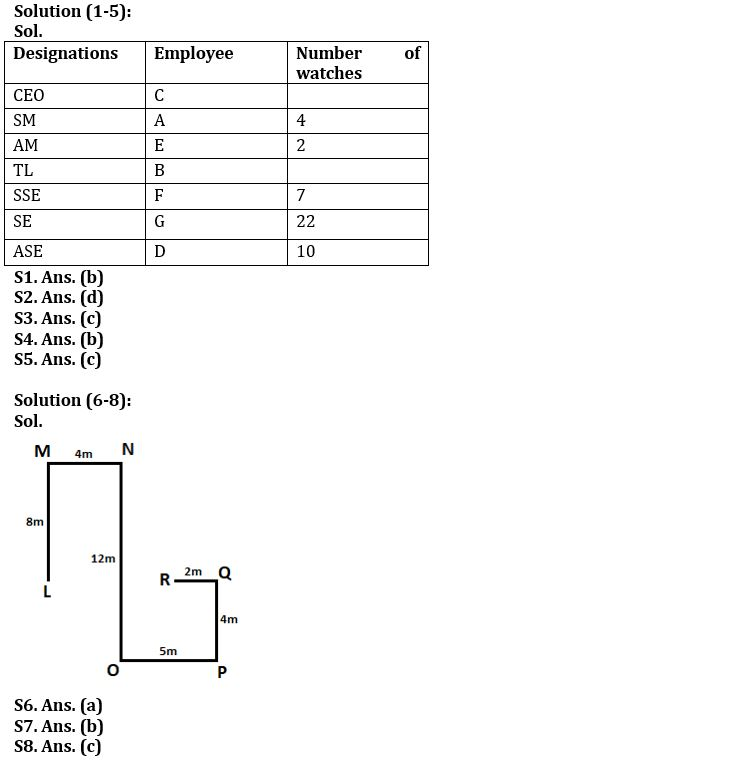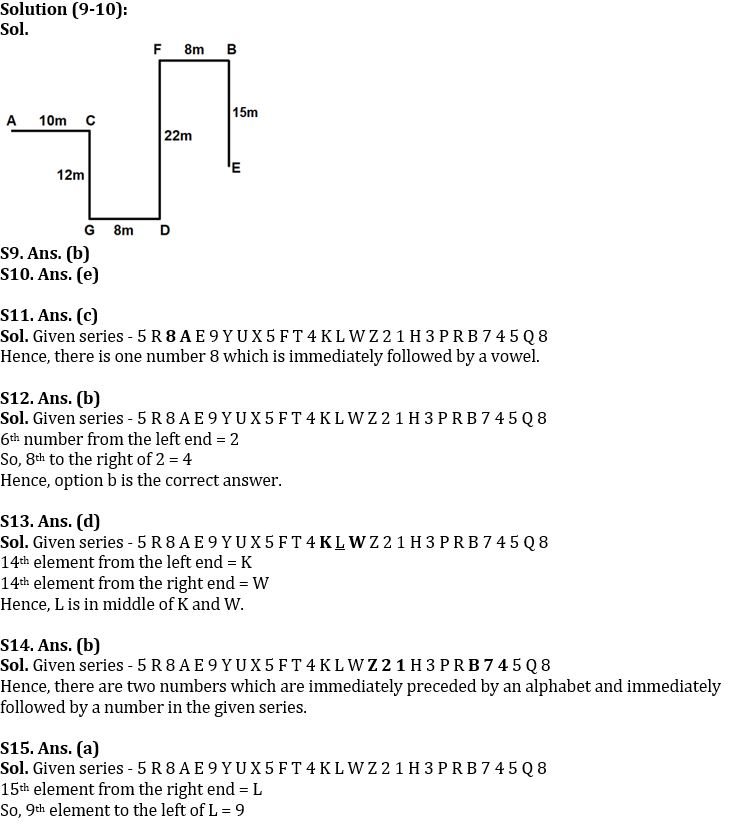Latest Banking jobs   »

# Reasoning Ability Quiz For IBPS Clerk/PO Prelims 2022- 15th August

Directions (1-5): Answer the questions based on the information given below.
Seven employees (A, B, C, D, E, F and G) of a company have different designations among- CEO, SM, AM, TL, SSE, SE and ASE, such that CEO is the senior most designation and ASE is the junior most designation. Five employees have different number (2, 4, 7, 10 and 22) of watches with them.
E who is AM, has 2 watches less than A. A is four designations senior to G. D who has 3 watches more than F, is junior than G. C is three designations senior to B. SM does not have odd number of watches. Difference between the number of watches of SE and ASE is more than 6.

Q1. Who is the CEO?
(a) A
(b) C
(c) F
(d) G
(e) None of these

Q2. How many persons are senior than F?
(a) One
(b) Two
(c) Three
(d) Four
(e) None of these

Q3. What is the sum of the number of watches of SM and SE?
(a) 14
(b) 24
(c) 26
(d) 29
(e) None of these

Q4. How many persons are senior than B?
(a) One
(b) Three
(c) Five
(d) Six
(e) None of these

Q5. F and G are ___ and _____ respectively.
(a) SM, SSE
(b) AM, SE
(c) SSE, SE
(d) SE, ASE
(e) None of these

Directions (6-8): Answer the questions based on the information given below.
Point M is 8m north of point L and 4m west of point N. Point O is 12m south of point N and 5m west of point P. Point Q is 4m north of point P and 2m east of point R.

Q6. What is the direction of Q with respect to L?
(a) East
(b) West
(c) North-west
(d) South-east
(e) None of these

Q7. What is the shortest distance between R and L?
(a) 6m
(b) 7m
(c) 8m
(d) 9m
(e) None of these

Q8. What is the direction of M with respect to P?
(a) North-east
(b) South-west
(c) North-west
(d) South-east
(e) None of these

Directions (9-10): Answer the questions based on the information given below.
A is 10m west of C. G is 12m south of C. D is 8m east of G. F is 22m north of D. B is 8m east of F. E is 15m south of B.

Q9. What is the direction of E with respect to A?
(a) East
(b) South-east
(c) North-west
(d) West
(e) South-west

Q10. What is the direction of G with respect to B?
(a) North -east
(b) South-east
(c) North-west
(d) West
(e) South-west

Directions (11-15): Study the following arrangement of numbers, symbols and letters carefully and answer the questions:

5 R 8 A E 9 Y U X 5 F T 4 K L W Z 2 1 H 3 P R B 7 4 5 Q 8

Q11. In the given series, how many numbers are immediately followed by a vowel?
(a) Two
(b) Three
(c) One
(d) Four
(e) None of these

Q12. In the given series, which of the following element is 8th to the right of 6th number from the left end?
(a) X
(b) 4
(c) B
(d) 3
(e) None of these

Q13. Which element is exactly in the middle of 14th element from left end and 14th element from right end?
(a) X
(b) Z
(c) W
(d) L
(e) None of these

Q14. How many numbers are immediately preceded by an alphabet and immediately followed by a number in the given series?
(a) One
(b) Two
(c) Three
(d) Four
(e) None of these

Q15. Which of the following element is 9th to the left of 15th element from the right end in the given series?
(a) 9
(b) E
(c) Y
(d) 8
(e) None of these

Solutions#### Congratulations!Download Hindu Review of October 2021: Free PDF